# Fraser diagram

(diff) ← Older revision | Latest revision (diff) | Newer revision → (diff)

A method for obtaining interpolation formulas with respect to the nodes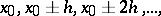at the point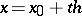, based on the relations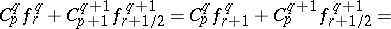whereare the finite differences of the functionand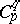are the binomial coefficients. For each path going from an arbitrary element of the left-hand column along the sides or horizontal diagonals of the rhombi in the Fraser diagram there is some corresponding interpolation formula. To obtain it one must follow the following rules.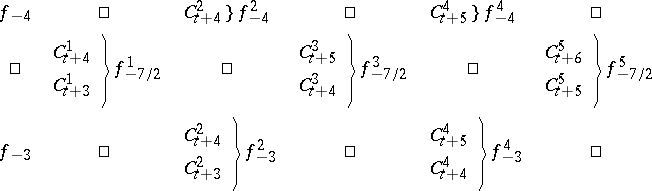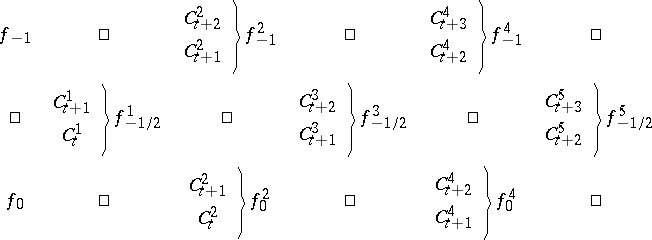1) When a column of differences is intersected from the left to the right, one term is added.

2) If the path comes in (from the left) to some column of differences along a side of a rhombus, then the added term is equal to the product of the difference at the intersection of the path and the column by the coefficient associated with the side of the rhombus that has been traversed.

3) If the path comes in (from the left) to some column of differences along a horizontal diagonal of a rhombus, then the added term is equal to the product of the difference at the intersection of the path and the column by the half-sum of the coefficients associated with the sides that come in (from the left) to the same vertex of the rhombus.

4) If the path intersects the column of differences (from the left to the right) along a horizontal diagonal of a rhombus between two differences, then one adds the product of the half-sum of these differences by the coefficient associated with the side of the rhombus immediately above (or below) the section of the diagonal that has been traversed.

5) Each part of a path that goes from the right to the left gives rise to the same terms as for going from the left to the right, but with the opposite sign.

6) One can deal with the column of tabular values of the function as the column of differences of order zero, by the same rules as with the other columns of differences.

How to Cite This Entry:
Fraser diagram. Encyclopedia of Mathematics. URL: http://encyclopediaofmath.org/index.php?title=Fraser_diagram&oldid=17389
This article was adapted from an original article by M.K. Samarin (originator), which appeared in Encyclopedia of Mathematics - ISBN 1402006098. See original article